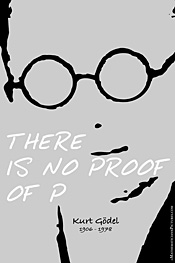home

## Gödels incompleteness theorem

In 1931 the Austrian mathematician Kurt Gödel published his famous "Über formal unentscheidbare Sätze der Principia Mathematica und verwandter Systeme I" ( Monatsheft für Math. und Physik 38, 1931, p.173-198) in which he could prove that any formal system which contains arithmetics (i.e. the mathematics of whole numbers) is incomplete. By incomplete is meant, that the system contains undecidable statements, i.e. statements which are neither provable nor disprovable (by means of the system itself).

More precisely Gödel showed, that the consistency of such a system implies the exsistence of undecidable statements (first incompleteness theorem) and that the consistency of the system itself is undecidable (second incompleteness theorem). By consistency is meant, that it is excluded to prove a statement together with its negation.

### Historical context

In order to appreciate this result it is necessary to put it into the context of the prevailing attitude towards the foundation of mathematics around 1900. A formative event was the emergence of antinomies (i.e. paradoxes) in e.g. set theory. The most famous of this antinomies is due to Bertrand Russel (mentioned by Russel in a letter to Frege 1902) and considers the set R which is defined as R={A | A∉ A}, i.e. the set of all sets which are not members of themselves. However, is R itself contained in R? If R∈R holds it needs to be excluded from R by definition. But if R∉R is assumed it needs to be contained! Thus the statements R∈R (=S) and R∉R (=¬S) imply each other. The significance of Russell's paradox can be seen once it is realized that, using classical logic, all sentences follow from a contradiction, i.e. the simultaneous validity of a statement, S, and its negation, ¬S. The argument is simple: from ¬S we obtain ¬S ∨ Q, with Q an arbitrary proposition. However, ¬S ∨ Q is equivalent to S⇒Q. Hence, given the simultaneous validity of S we have derived the arbitrary proposition Q!

It became apparent that utmost accuracy is needed in order to avoid such contradictory results. The Russel antinomy stems from the idea that any coherent condition may be used to determine a set. As a result, most attempts at resolving the paradox have concentrated on various ways of restricting the principles governing set existence, i.e. to refine the axioms of set theory. One such suggestion was developed by Ernst Zermelo since 1908 (later extended by Abraham Fraenkel). Within the Zermelo-Fraenkel set theory the object R discussed above cannot be constructed. However, even at this stage it became clear that the original goal of logicism to base all of mathematics on logic (plus evident axioms) only could not be carried out because the extra axioms needed to prevent the antinomies to sneak in were not "logical" or "self evident" - at least in the narrow sense.

### Hilbert's program

However, worse was still to come! The question was: what reason does one have to believe that e.g. the Zermelo-Fraenkel axiomatic system can not give rise to other yet undiscovered paradoxes? Certainly a proof of the consistency of a given axiomatic system would be more than welcome! Presumably the most influential figure within this debate was David Hilbert who championed the axiomatic method. He could for example trace back the consistency of his axiomatization of geometry to the consistency of arithmetics. In 1900 he delivered an influential speech at the International Congress of Mathematicians in Paris in which he formulated open problems of mathematical research. The published version contained 23 problems, and the second on this list was the consistency proof of the axioms of arithmetics.

"Vor allem aber möchte ich unter den zahlreichen Fragen, welche hinsichtlich der Axiome gestellt werden können, dies als das wichtigste Problem bezeichnen, zu beweisen, daß dieselben untereinander widerspruchslos sind, d.h. daß man auf Grund derselben mittelst einer endlichen Anzahl von logischen Schlüssen niemals zu Resultaten gelangen kann, die miteinander in Widerspruch stehen." (Hilbert, 1900)

Apparently this goal was proven unobtainable by Gödel in 1931. The links below direct to sources which (i) provide a more technical understanding of the theorem and its proof and (ii) investigate the implications of this discovery.

To simplify the original Gödel proof has become an industry. One of the earliest attempts in doing so is due to Rosser (1939). An excellent discussion of the implications is given in Goodstein (1963). An other neat summary of the history, proof and related matters can be found in Zach. I also do like this one by Robert Low.

Gödel simplified